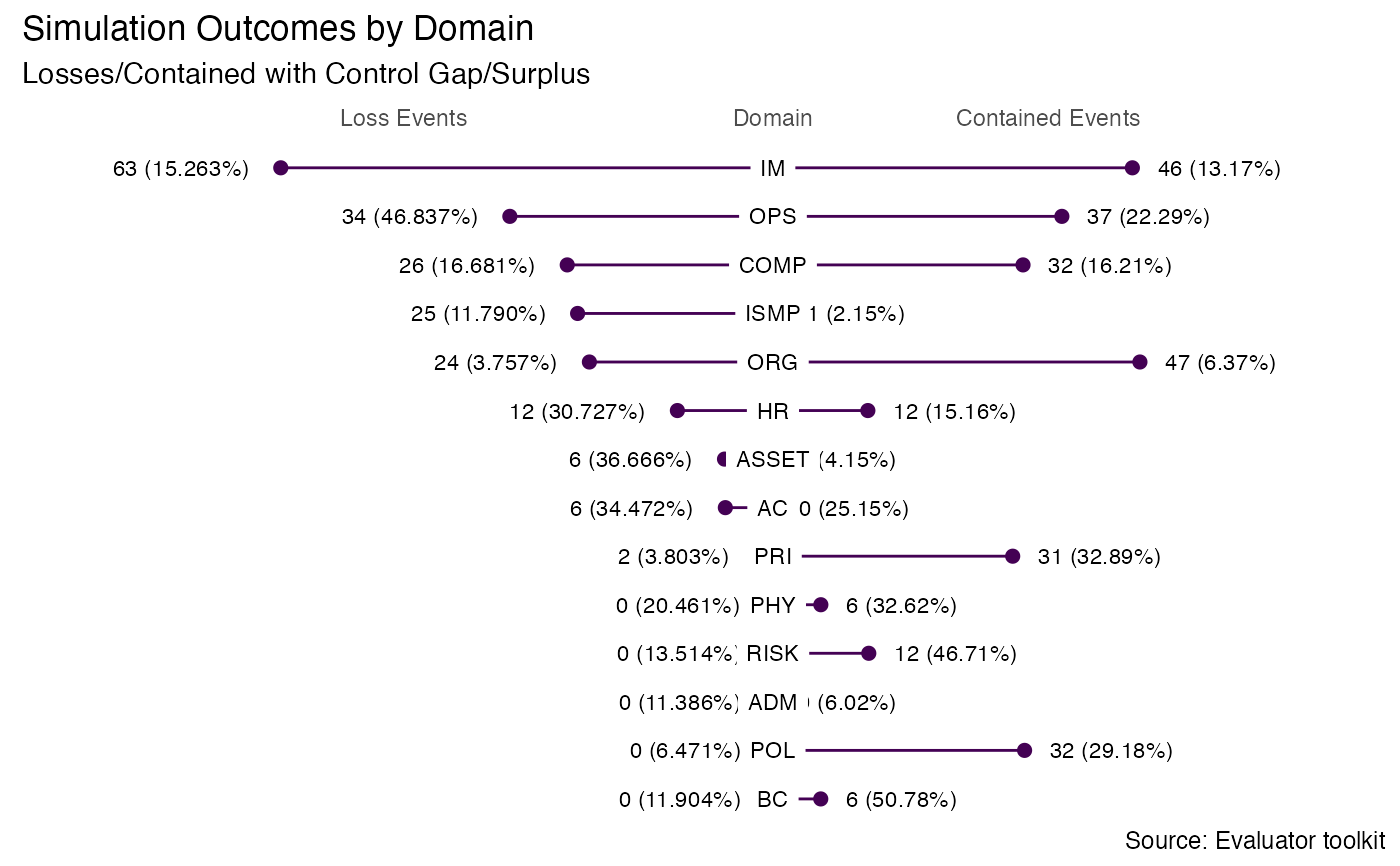Creates a barbell plot showing the number and percentage of events contained (not resulting in loss) vs the number and percentage of loss events (threat events resulting in losses).

generate_event_outcomes_plot(domain_summary, domain_id = domain_id)

## Arguments

domain_summary

Domain-level summary from domain_summary.

domain_id

Variable to group plot by.

## Value

A ggplot object.

Other result graphs: exposure_histogram(), generate_heatmap(), generate_scatterplot-deprecated, loss_exceedance_curve(), loss_scatterplot()
data(mc_domain_summary)